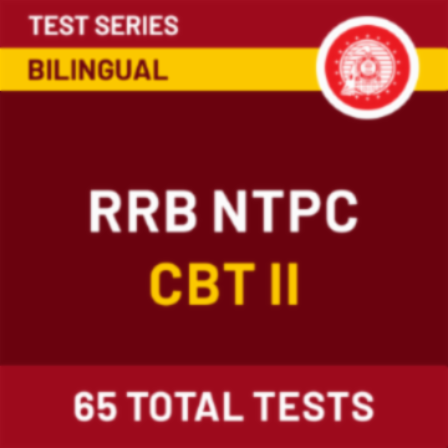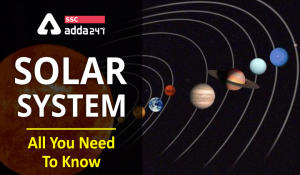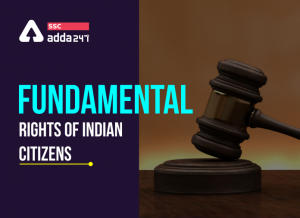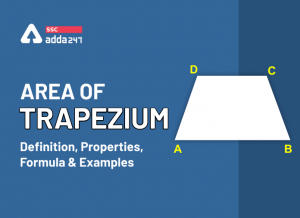Latest SSC jobs   »   REFLECTION OF LIGHT

# Reflection of Light: Definition, Types, Formulas

Dear Readers, GA section is taken on the light note but plays a vital role in achieving the score required to cross the cut-off marks. We are providing important Notes for this, it will help you to score well in GA section if you regularly follow the contents provided on scaffold website and Adda247 app regarding general knowledge important questions. We wish you the best wishes for all the upcoming exams.

## REFLECTION OF LIGHT

A highly polished surface, such as a mirror, reflects most of the light falling on it. The phenomenon of bouncing back of light from a surface is known as the reflection of light. An object reflects light that falls on it. This reflected light, when received by our eyes, enables us to see things. We are able to see through a transparent medium as light is transmitted through it.

The laws of reflection of light-
(i)The angle of incidence is equal to the angle of reflection,
(ii)The incident ray, the normal to the mirror at the point of incidence and the reflected ray, all lie in the same plane. These laws of reflection are applicable to all types of reflecting surfaces including spherical surfaces.

## SPHERICAL MIRRORS

The reflecting surface of a spherical mirror may be curved inwards or outwards.
For spherical mirrors of small apertures, the radius of curvature is found to be equal to twice the focal length i.e. R = 2f. This implies that the principal focus of a spherical mirror lies midway between the pole and centre of curvature.

### 1.Concave mirror

A spherical mirror, whose reflecting surface is curved inwards, that is, faces towards the centre of the sphere, is called a concave mirror.

Image formation by a concave mirrorUses of concave mirrors

•  Used in torches, search-lights and vehicles headlights to get powerful parallel beams of light.
• As shaving mirrors to see a larger image of the face.
• The dentists use concave mirrors to see large images of the teeth of patients.
• Large concave mirrors are used to concentrate sunlight to produce heat in solar furnaces.

### 2.Convex mirror

A spherical mirror whose reflecting surface is curved outwards, is called a convex mirror.

Image formation by a convex mirrorUses of a convex mirror

• Commonly used as rear-view (wing) mirrors in vehicles. These mirrors are fitted on the sides of the vehicle, enabling the driver to see traffic behind him/her to facilitate safe driving. It enables the driver to view much larger area than would be possible with a plane mirror.
• In big showrooms and departmental stores, convex mirrors are used to have a view on the customers entering in as well as going out.

Important terms-

Ray of Light : A line drawn in the direction of propagation of light is called a ray of light.

Beam of Light : A group of rays of light emitted by a source of light is called a beam of light. A light beam is of three types.

Real Image : It is a kind of image which is formed by actual intersection of light rays after reflection.

Virtual Image : It is a kind of image which is formed by producing the reflected rays backward after reflection.

Pole: The centre of the reflecting surface of a spherical mirror is a point called the pole. It lies on the surface of the mirror.

Centre of curvature: The reflecting surface of a spherical mirror forms a part of a sphere. This sphere has a centre. This point is called the centre of curvature of the spherical mirror.

Radius of curvature: The radius of the sphere of which the reflecting surface of a spherical mirror forms a part, is called the radius of curvature of the mirror.

Principal axis: A straight line passes through the pole and the centre of curvature of a spherical mirror. This line is called the principal axis.

Principal Focus: When rays from infinity come in parallel to the optical axis of a spherical mirror, they are bent so that they either converge and intersect in at a point, or they seem to diverge from a point. The point of convergence or divergence is called the focus. It is denoted by letter F.

Focal length: The distance between the pole and the principal focus of a spherical mirror is called the focal length. It is represented by the letter f.

Aperture: The diameter of the reflecting surface of spherical mirror is called its aperture.

Magnification: Magnification produced by a spherical mirror gives the relative extent to which the image of an object is magnified with respect to the object size.

If h is the height of the object and h′ is the height of the image, then the magnification m produced by a spherical mirror is given by:

The magnification m is also related to the object distance (u) and image distance (v):

## MIRROR FORMULA

In a spherical mirror, the distance of the object from its pole is called the object distance (u). The distance of the image from the pole of the mirror is called the image distance (v). The distance of the principal focus from the pole is called the focal length (f).  There is a relationship between these three quantities given by the mirror formula which is expressed as-
Sign Convention for Reflection by Spherical Mirrors
While dealing with the reflection of light by spherical mirrors, we shall follow a set of sign conventions called the New Cartesian Sign Convention. In this convention, the pole (P) of the mirror is taken as the origin. The principal axis of the mirror is taken as the x-axis (X’X) of the coordinate system. The conventions are as follows –
(i)The object is always placed to the left of the mirror. This implies that the light from the object falls on the mirror from the left-hand side.
(ii) All distances parallel to the principal axis are measured from the pole of the mirror.
(iii)All the distances measured to the right of the origin (along + x-axis) are taken as positive while those measured to the left of the origin (along – x-axis) are taken as negative.
(iv)Distances measured perpendicular to and above the principal axis (along + y-axis) are taken as positive.
(v)Distances measured perpendicular to and below the principal axis (along –y-axis) are taken as negative.
Questions related to it-
Q.1 A convex mirror used for rear-view on an automobile has a radius of curvature of 3.00 m. If a bus is located at 5.00 m from this mirror, find the position, nature and size of the image.
Solution-
Q.2 The image formed by a concave mirror is observed to be virtual, erect and larger than the object. Where should be the position of the object?
(a)Between the principal focus and the centre of curvature
(b) At the centre of curvature
(c) Beyond the centre of curvature
(d) Between the pole of the mirror and its principal focus.
Ans D
Q.3 No matter how far you stand from a mirror, your image appears erect. The mirror is likely to be:
(a)plane.
(b)concave.
(c)convex.
(d)either plane or convex.
Ans D
Q.4 A concave mirror produces three times magnified (enlarged) real image of an object placed at 10 cm in front of it. Where is the image located?
Solution:
Let the height of the object= h
Then,the height of the image= -3h (real image)
m= -3h/h = -v/u
v/u will be 3
As, u = -10cm (given)
v = 3 x (-10) = -30cm
Hence, an inverted image is formed at a distance of 30cm in front of the given concave mirror.

###### Congratulations!

General Awareness & Science Capsule PDF

Incorrect details? Fill the form again here

General Awareness & Science Capsule PDF

Thank You, Your details have been submitted we will get back to you.
•Mensuration Formula And Questions For 2D...
•Our Solar System, Formation, Planets, Fa...
•Fundamental Rights Of Indian Citizens: D...
•Study Notes On "BLOOD" For SSC & Rai...
•Important Notes on Mughal Empire: Rulers...
•Area Of Trapezium: Definition, Propertie...